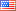# Revision history [back]

### How to expand an symbolic expression.

With Maple I can write T := proc(f,n,x) local v; add(binomial(n,v)f(v+1)(x)^v,v=0..n) end: and then T(f,4,x) will give me the answer f(1)+4f(2)x+6f(3)x^2+4f(4)x^3+f(5)*x^4.

If I write the same with Sage def T(f,n,x) : return add(binomial(n,v)f(v+1)(x)^v for v in (0..n)) var('f') T(f,4,x) then I will get the answer 5x^4 + 16x^3 + 18x^2 + 8x + 1.

How do I have to proceed to get Maple's answer?2 No.2 RevisionShashank1887 ●28 ●47 ●82

### How to expand an symbolic expression.

With Maple I can write

T := proc(f,n,x)  local v; add(binomial(n,v)f(v+1)(x)^v,v=0..n)
end:


and then T(f,4,x)

T(f,4,x)


will give me the answer f(1)+4f(2)x+6f(3)x^2+4f(4)x^3+f(5)*x^4.

f(1)+4*f(2)*x+6*f(3)*x^2+4*f(4)*x^3+f(5)*x^4.


If I write the same with Sage

def T(f,n,x) : T(f,n,x):

5*x^4 + 16x^3 16*x^3 + 18x^2 18*x^2 + 8x 8*x + 1.1.